Suppose the mean percentage in Algebra 2B is 70% and the standard deviation is 8% What percentage of students receive between a 70% and 94%

Question

Suppose the mean percentage in Algebra 2B is 70% and the standard deviation is 8% What percentage of students receive between a 70% and 94% enter the value of the percentage without the percent sign

in progress 0
5 months 2021-08-27T13:10:12+00:00 1 Answers 4 views 0

49.87

Step-by-step explanation:

Normal Probability Distribution:

Problems of normal distributions can be solved using the z-score formula.

In a set with mean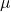and standard deviation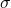, the z-score of a measure X is given by: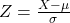The Z-score measures how many standard deviations the measure is from the mean. After finding the Z-score, we look at the z-score table and find the p-value associated with this z-score. This p-value is the probability that the value of the measure is smaller than X, that is, the percentile of X. Subtracting 1 by the p-value, we get the probability that the value of the measure is greater than X.

Suppose the mean percentage in Algebra 2B is 70% and the standard deviation is 8%.

This means that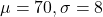What percentage of students receive between a 70% and 94%

The proportion is the p-value of Z when X = 94 subtracted by the p-value of Z when X = 70. So

X = 94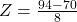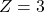has a p-value of 0.9987.

X = 70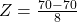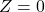has a p-value of 0.5.

0.9987 – 0.5 = 0.4987.

0.4987*100% = 49.87%.

So the percentage is 49.87%, and the answer, without the percent sign, is 49.87.